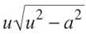﻿ ﻿INTEGRATING INVERSE TRIGONOMETRIC FUNCTIONS - Integration - AP CALCULUS AB & BC REVIEW - Master AP Calculus AB & BC

## Master AP Calculus AB & BC

Part II. AP CALCULUS AB & BC REVIEW

CHAPTER 7. Integration

INTEGRATING INVERSE TRIGONOMETRIC FUNCTIONS

You already know a lot about integrating inverse trigonometric functions. In fact, you know so much that your parents pull pictures of you from their wallet and drone on and on about your intimate knowledge of arcsin. In fact, they wish you were going out with arcsin instead of that no-good you’re currently dating. Well, it’s time to give them even more to brag about.

You already know thatHowever, a radical in the denominator does not have to contain a 1, and the squared term does not have to be x2. You can integrate any expression of the formto get arcsin u/a (where a is a constant and u is a function of x).

Example 15: EvaluateSolution: First of all, pull that 5 out of the numerator. The numerator has to be 1, according to the formula above. Don’t worry about the presence of the x for now... . That will take care of itself later.We still have the arcsin formin the denominator; a2 = 9 and u2 = x4. Therefore, a = 3 and u = x2. The presence of a u reminds you to do usubstitution.

u = x2

du = 2x dx

Therefore, du/2 will replace the xdx in the denominator when we substitute. (See? I told you the x would take care of itself.)According to the formula above, this equals arcsin u/a, which isNOTE. You don’t have to memorize a pattern for arccosx, arccot x, or arccsc x, since their patterns differ only by a negative sign (remember from the derivatives section?). Don’t even worry about using them.

That’s all there is to it. Recognize the pattern ofin the denominator, and it’s a good clue to try to integrate using arcsin x. However, there are two other patterns you want to memorize as well (not five, as you might have feared). Here are the remaining two (and they look remarkably similar to the derivatives you found earlier in the book).Pattern to look for: The sum of a number and a variable to a power in the denominator.Pattern to look for: A radical in the denominator and the difference of a variable to a power and a number. Arcsec is very close to arcsin, but the order of the subtraction is reversed—not to mention the presence of that extra x in the arcsec formula.

Both of these formulas have a 1/a in front of the inverse trigonometric formula, whereas arcsin x does not. It does not change the procedure at all; just don’t forget it.

Example 16: Integrate the following:This is a job for arcsec. Thepattern is evident in the denominator: u = x3 and a = 2. Don’t forget—the u reminds you to do u-substitution. If u = x3, then du = 3x2 and du/3 = x2. This gives youThis one may not look like arctan, but it is. The denominator has the form u2 + a2, where u = cos x and a = √3. The constant does not have to be a perfect square. This works exactly the same way. Don’t forget about u-substitution, though. If u = cos x, du = —sin x, and —du = sin x.You can rationalize this, if you wish, to getEXERCISE 8

Directions: Solve each of the following problems. Decide which is the best of the choices given and indicate your responses in the book.

EVALUATE EACH OF THE FOLLOWING WITHOUT A CALCULATOR1. This is a clear arctan problem with u = 2x and a = √7. Therefore, du = 2dx and du/2 = dx.2. You cannot use inverse trig formulas to solve this. If you tried, you would have set u = 1/√x, but the resulting u-substitution would have been impossible—you’d need another 1/√x in the problem. Instead, this is a simple «-substitution problem. Set u = x + 7 and du = dx. You can then rewrite the integral aswhich is simply3. This is definitely an arcsin problem, but it’s much easier if you factor out a 16 from the denominator and simplify first.This could hardly be more straightforward now. Set u = x and a = 2, so du = dx and the integral becomes4. Your instinct should tell you that this is an arcsec problem, since there is a radical in the denominator and the order of subtraction is variable — constant. However, if u = cot x, shouldn’t there be a cot x in front of the radical to match the correct denominator form ofWatch what happens when you rewrite the trig functions using the reciprocal identities:Now, if u = cot x (and a = 4), du = —csc2x dx, so —du = csc2x dx. Rewrite the integral to getwhich is the exact formula for arcsec, and everybody’s happy.﻿